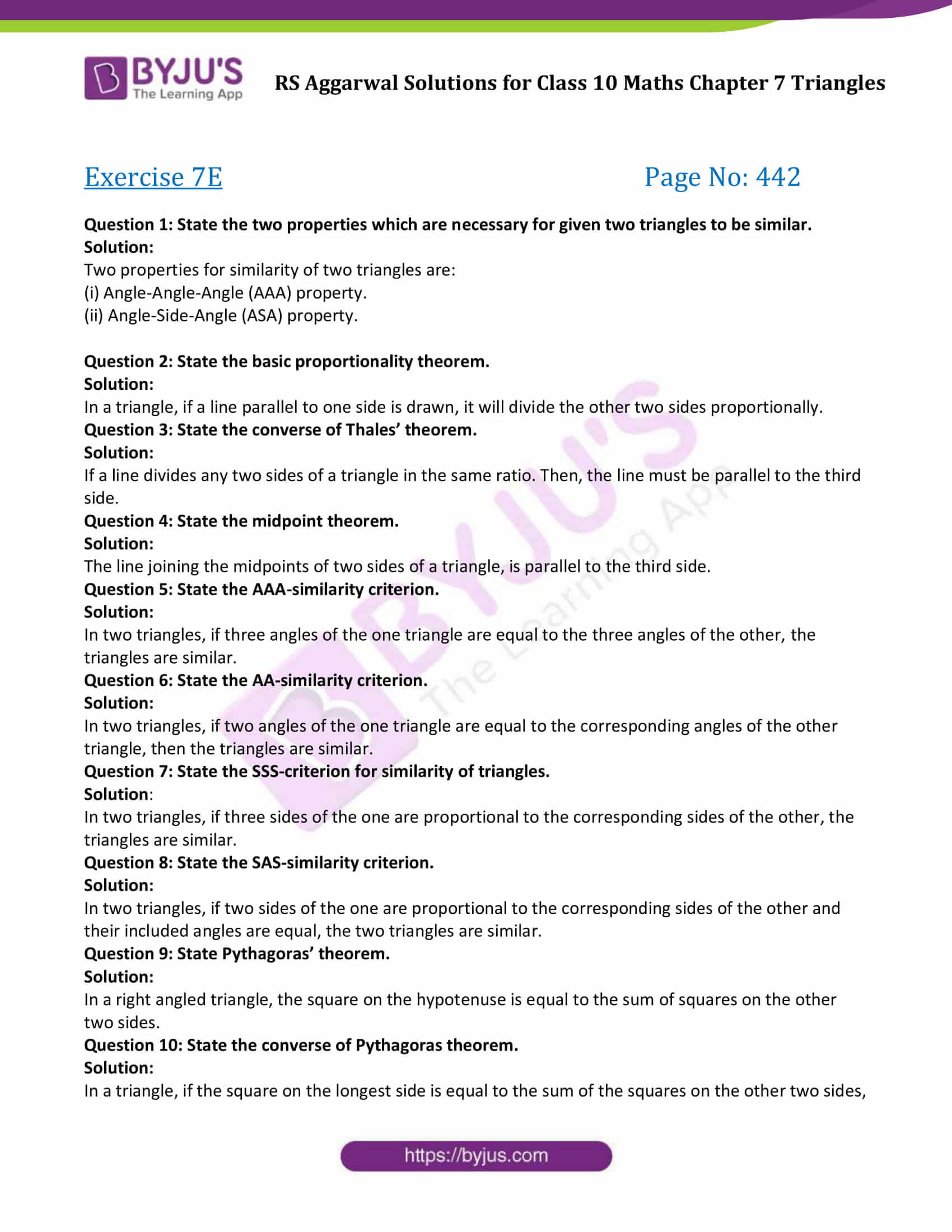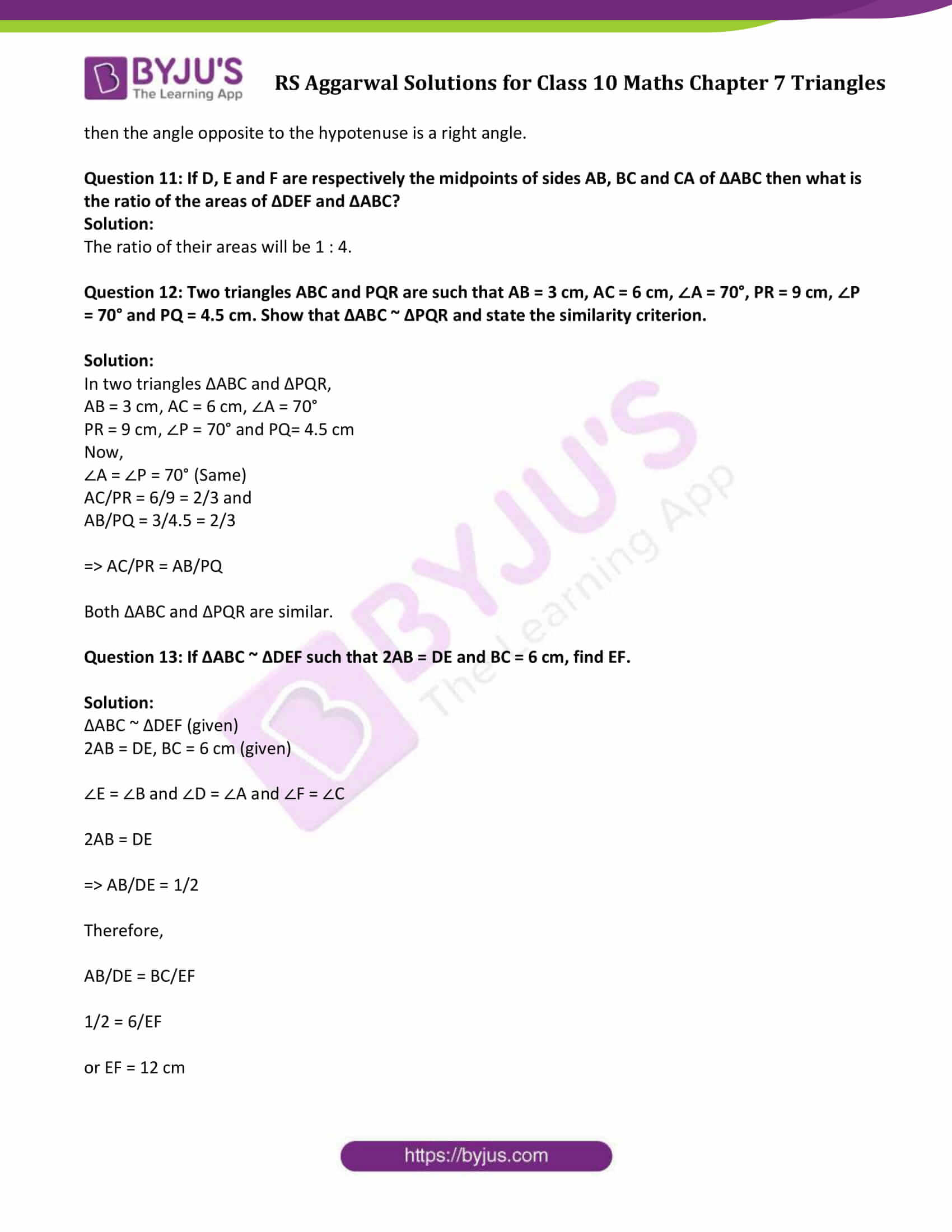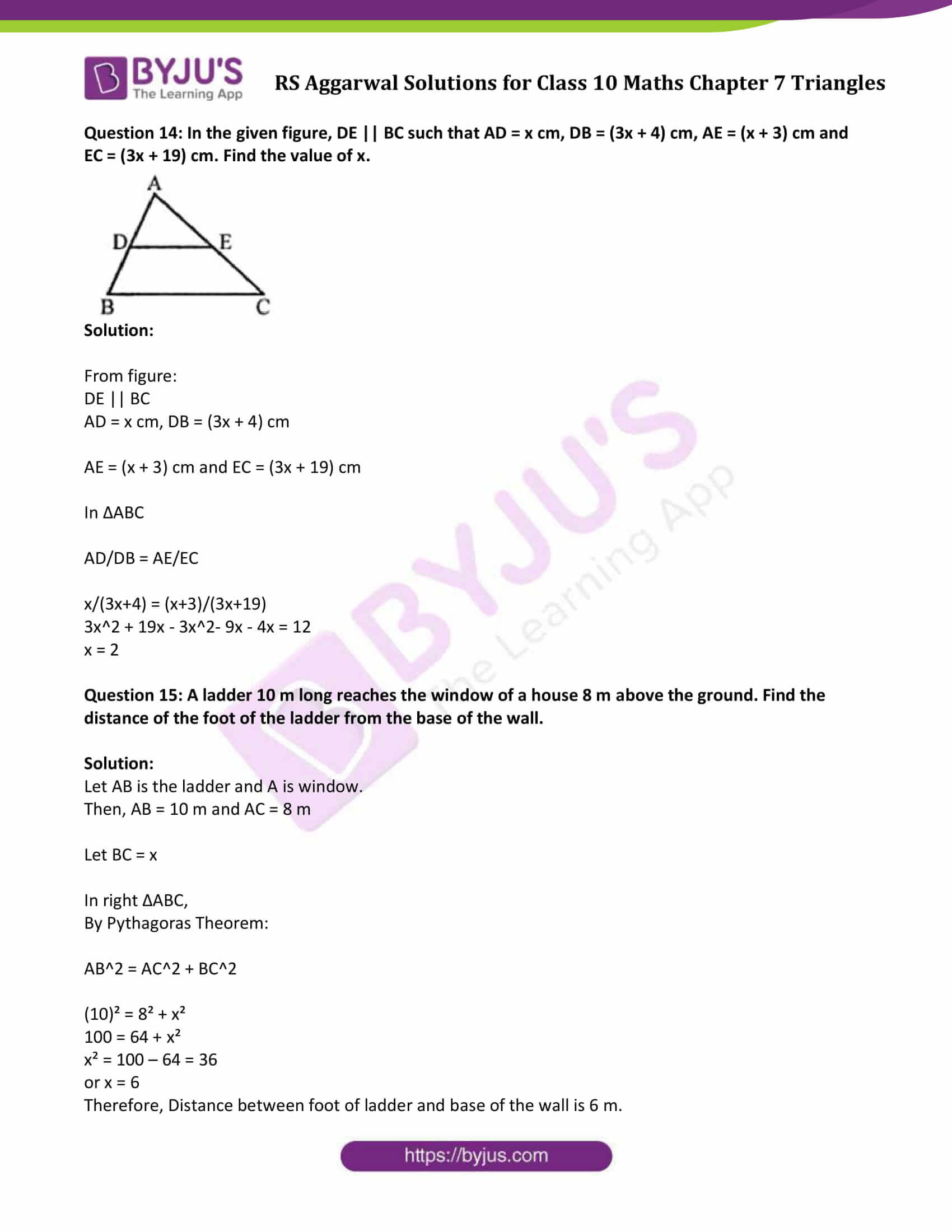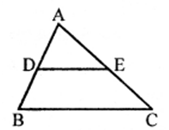# R S Aggarwal Solutions for Class 10 Maths Chapter 7 Triangles Exercise 7E

R S Aggarwal Solutions for Class 10 Maths helps students develop a strong foundational base for all the important concepts. R S Aggarwal Solutions for class 10 Maths chapter 7 exercise 7E is available here. These solutions are available in PDF format and students can freely download it by clicking on the click below.

## Download PDF of R S Aggarwal Solutions for Class 10 Maths Chapter 7 Triangles Exercise 7E### Access other exercise solutions of Class 10 Maths Chapter 7 Triangles

Exercise 7 A Solutions

Exercise 7 B Solutions

Exercise 7 C Solutions

Exercise 7 D Solutions

Question 1: State the two properties which are necessary for given two triangles to be similar.

Solution:

Two properties for similarity of two triangles are:

(i) Angle-Angle-Angle (AAA) property.

(ii) Angle-Side-Angle (ASA) property.

Question 2: State the basic proportionality theorem.

Solution:

In a triangle, if a line parallel to one side is drawn, it will divide the other two sides proportionally.

Question 3: State the converse of Thales’ theorem.

Solution:

If a line divides any two sides of a triangle in the same ratio. Then, the line must be parallel to the third side.

Question 4: State the midpoint theorem.

Solution:

The line joining the midpoints of two sides of a triangle, is parallel to the third side.

Question 5: State the AAA-similarity criterion.

Solution:

In two triangles, if three angles of the one triangle are equal to the three angles of the other, the triangles are similar.

Question 6: State the AA-similarity criterion.

Solution:

In two triangles, if two angles of the one triangle are equal to the corresponding angles of the other triangle, then the triangles are similar.

Question 7: State the SSS-criterion for similarity of triangles.

Solution:

In two triangles, if three sides of the one are proportional to the corresponding sides of the other, the triangles are similar.

Question 8: State the SAS-similarity criterion.

Solution:

In two triangles, if two sides of the one are proportional to the corresponding sides of the other and their included angles are equal, the two triangles are similar.

Question 9: State Pythagoras’ theorem.

Solution:

In a right angled triangle, the square on the hypotenuse is equal to the sum of squares on the other two sides.

Question 10: State the converse of Pythagoras theorem.

Solution:

In a triangle, if the square on the longest side is equal to the sum of the squares on the other two sides, then the angle opposite to the hypotenuse is a right angle.

Question 11: If D, E and F are respectively the midpoints of sides AB, BC and CA of ∆ABC then what is the ratio of the areas of ∆DEF and ∆ABC?

Solution:

The ratio of their areas will be 1 : 4.

Question 12: Two triangles ABC and PQR are such that AB = 3 cm, AC = 6 cm, ∠A = 70°, PR = 9 cm, ∠P = 70° and PQ = 4.5 cm. Show that ∆ABC ~ ∆PQR and state the similarity criterion.

Solution:

In two triangles ∆ABC and ∆PQR,

AB = 3 cm, AC = 6 cm, ∠A = 70°

PR = 9 cm, ∠P = 70° and PQ= 4.5 cm

Now,

∠A = ∠P = 70° (Same)

AC/PR = 6/9 = 2/3 and

AB/PQ = 3/4.5 = 2/3

=> AC/PR = AB/PQ

Both ∆ABC and ∆PQR are similar.

Question 13: If ∆ABC ~ ∆DEF such that 2AB = DE and BC = 6 cm, find EF.

Solution:

∆ABC ~ ∆DEF (given)

2AB = DE, BC = 6 cm (given)

∠E = ∠B and ∠D = ∠A and ∠F = ∠C

2AB = DE

=> AB/DE = 1/2

Therefore,

AB/DE = BC/EF

1/2 = 6/EF

or EF = 12 cm

Question 14: In the given figure, DE || BC such that AD = x cm, DB = (3x + 4) cm, AE = (x + 3) cm and EC = (3x + 19) cm. Find the value of x.Solution:

From figure:

DE || BC

AD = x cm, DB = (3x + 4) cm

AE = (x + 3) cm and EC = (3x + 19) cm

In ∆ABC

x/(3x+4) = (x+3)/(3x+19)

3x2 + 19x – 3x2– 9x – 4x = 12

x = 2

Question 15: A ladder 10 m long reaches the window of a house 8 m above the ground. Find the distance of the foot of the ladder from the base of the wall.

Solution:

Let AB is the ladder and A is window.

Then, AB = 10 m and AC = 8 m

Let BC = x

In right ∆ABC,

By Pythagoras Theorem:

AB2 = AC2 + BC2

(10)² = 8² + x²

100 = 64 + x²

x² = 100 – 64 = 36

or x = 6

Therefore, Distance between foot of ladder and base of the wall is 6 m.

## R S Aggarwal Solutions for Class 10 Maths Chapter 7 Triangles Exercise 7E

Class 10 Maths Chapter 7 Triangles Exercise 7E is based on the topics:

• Similar Triangles
• Thales’ Theorem and it’s converse
• Midpoint theorem
• Similarity of two triangles
• Similarity of two triangles and its results.